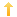## PowerBuilder – ‘Smart’ Crosstab Datawindow

Posted on Thursday, March 4th, 2010 at 8:20 pm in

I had a question from a co-worker regarding a functionality request from a user. Basically they wanted a datawindow to display information for vendors (basic stuff like name, amt 1, amt 2, amt 3, etc.) for a specific set of amount columns. Each line represents one vendor and the various amounts (if any) with a total for the line. Each column of the amounts would be totaled at the bottom. Classic ‘cross tab’ type stuff.

However, the users only wanted an amount column to display if it had a value in it, i.e., if no vendor had this amount, don’t put it on the datawindow. The other request was to have one specific column to not be totaled with the others for each vendor, i.e., the line total was the total of all the other amounts except a specified one. We have now left the ‘cross tab’ universe.

My Solution

As a proof of concept I created a Grid Style, external datawindow with five specified columns. Column one is the vendor name; the other four are numeric (I called them box1 through box4). I then added a series of data values (vendor name and numbers). I made sure that one column of amounts was blank ( or had a value of zero).
To handle the requirement of totaling all amounts except one specified, I created a computed field at the end of the others. The expression is:

`if(isnull(box1),0,box1) + if(isnull(box2),0,box2) + if(isnull(box3),0,box3)`

In this case I want to exclude box4 from the total.
I also created a series of totals for each column (using the painter to generate the expression).

The final step was to create the code which will dynamically hide any of the amount columns which have no values (or just zero amounts). The ‘workhorse’ of this code is the use of a describe to evaluate an expression on each row to determine if it has a value other than zero.

Code from Open event of window

```long        ll_i, ll_max, ll_x
long ll_basearraycount
string ls_basevisibles[], ls_basewidths[], ls_makevisible[]
string ls_colname, ls_coltype,  ls_msg

dw_1.retrieve( )

dw_1.setredraw( False)

// get list of columns in the order they are displayed which may not be the same as their column number order
ll_max = Integer(dw_1.Object.DataWindow.Column.Count)  // determine number of columns
FOR ll_i = 1 TO ll_max
// only need the visible columns
IF dw_1.describe("#" + String(ll_i) + ".Visible") = '1' THEN
ll_basearraycount ++
ls_basevisibles[ll_basearraycount] = dw_1.describe( "#" + string(ll_i) +  ".Name")
ls_basewidths[ll_basearraycount] = dw_1.describe( "#" + string(ll_i) +  ".Width")

ls_coltype = dw_1.describe( "#" + string(ll_i) +  '.ColType')

If (UPPER(ls_coltype) = 'NUMBER') THEN
// this gives a sum which means the column has data in it, ie, we want it visible
ls_msg = dw_1.describe( "evaluate ( 'sum(" + "#" + string(ll_i) + " for all)' , 1)  " )
IF Dec(ls_msg) <> 0 and not IsNull(Dec(ls_msg)) THEN
ls_makevisible[ll_basearraycount] = 'Y'
END IF
ELSE
IF ls_basevisibles[ll_basearraycount] = 'vendor' THEN // the first column is always visible
ls_makevisible[ll_basearraycount] = 'Y'
END IF
END IF

END IF
NEXT

//              Now make all columns invisible...
FOR ll_i = 1 TO ll_max
dw_1.Modify("#" + String(ll_i) + ".Visible='0'") // make it invisible
NEXT
// Now make the columns with values back visible
ll_max = UpperBound(ls_basevisibles)
ll_x = 10
FOR ll_i = 1 TO ll_max
IF ls_makevisible[ll_i] = 'Y' THEN
ls_msg = dw_1.Modify(ls_basevisibles[ll_i] + ".Visible='1'")          // make it visible
ls_msg = dw_1.Modify(ls_basevisibles[ll_i] + ".X=" + string(ll_x)) // set the X position (starts at 10)
ll_x +=Long(ls_basewidths[ll_i])   // keep track of running X position
ls_msg = dw_1.Modify(ls_basevisibles[ll_i] + ".Width=" + ls_basewidths[ll_i])     // set the column width
END IF
NEXT

dw_1.setRedraw(true)```

Sample code files below including export of objects (need PowerBuilder 11.5/12 or above to read pbl)
SmartCrosstab

You might also be interested in
Tags: